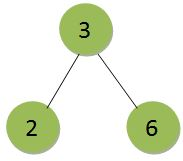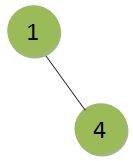# Generate two BSTs from the given array such that maximum height among them is minimum

Given an array of n integers, the task is to make two Binary Search Tree from the given array (in any order) such that the maximum height among the two trees is minimum possible and print the maximum height.

Examples:

Input: arr[] = {1, 2, 3, 4, 6}
Output: 1Input: arr[] = { 74, 25, 23, 1, 65, 2, 8, 99 }
Output: 2

## Recommended: Please try your approach on {IDE} first, before moving on to the solution.

Approach: The aim is to minimize the height of the maximum height binary search tree and to do so we need to divide the array elements equally among both the trees. And since the order doesn’t matter, we can easily choose any element for the first or second binary tree. Now, to minimize the height of the two tree, the trees will have to be almost complete and as equal in heights as possible. And the maximum (minimized) height will be log(n/2) or log(n/2 + 1).

Below is the implementation of the above approach:

## C++

 `// C++ implementation of the approach ` `#include ` `using` `namespace` `std; ` ` `  `// Function to calculate the log() ` `int` `cal_log(``int` `n) ` `{ ` `    ``int` `ans = 0; ` ` `  `    ``while` `(n) { ` `        ``n /= 2; ` `        ``ans++; ` `    ``} ` ` `  `    ``return` `ans; ` `} ` ` `  `// Function to return the maximum ` `// height of the tree ` `int` `maximumHeight(``int` `n, ``int` `arr[]) ` `{ ` `    ``int` `level = cal_log(n / 2 + n % 2); ` `    ``return` `(level - 1); ` `} ` ` `  `// Driven code ` `int` `main() ` `{ ` `    ``int` `arr[] = { 1, 2, 3, 4, 6 }; ` `    ``int` `n = ``sizeof``(arr) / ``sizeof``(arr); ` `    ``cout << maximumHeight(n, arr); ` ` `  `    ``return` `0; ` `} `

## Java

 `// Java implementation of the approach ` `import` `java.io.*; ` ` `  `class` `GFG ` `{ ` ` `  `// Function to calculate the log() ` `static` `int` `cal_log(``int` `n) ` `{ ` `    ``int` `ans = ``0``; ` ` `  `    ``while` `(n > ``0``)  ` `    ``{ ` `        ``n /= ``2``; ` `        ``ans++; ` `    ``} ` ` `  `    ``return` `ans; ` `} ` ` `  `// Function to return the maximum ` `// height of the tree ` `static` `int` `maximumHeight(``int` `n, ``int` `arr[]) ` `{ ` `    ``int` `level = cal_log(n / ``2` `+ n % ``2``); ` `    ``return` `(level - ``1``); ` `} ` ` `  `// Driver code ` `public` `static` `void` `main (String[] args)  ` `{ ` `    ``int` `arr[] = { ``1``, ``2``, ``3``, ``4``, ``6` `}; ` `    ``int` `n =arr.length; ` `    ``System.out.print( maximumHeight(n, arr)); ` `} ` `} ` ` `  `// This code is contributed by anuj_67.. `

## C#

 `// C# implementation of the approach ` `using` `System; ` ` `  `class` `GFG ` `{ ` ` `  `// Function to calculate the log() ` `static` `int` `cal_log(``int` `n) ` `{ ` `    ``int` `ans = 0; ` ` `  `    ``while` `(n > 0)  ` `    ``{ ` `        ``n /= 2; ` `        ``ans++; ` `    ``} ` ` `  `    ``return` `ans; ` `} ` ` `  `// Function to return the maximum ` `// height of the tree ` `static` `int` `maximumHeight(``int` `n, ``int` `[]arr) ` `{ ` `    ``int` `level = cal_log(n / 2 + n % 2); ` `    ``return` `(level - 1); ` `} ` ` `  `// Driver code ` `public` `static` `void` `Main ()  ` `{ ` `    ``int` `[]arr = { 1, 2, 3, 4, 6 }; ` `    ``int` `n =arr.Length; ` `    ``Console.WriteLine( maximumHeight(n, arr)); ` `} ` `} ` ` `  `// This code is contributed by anuj_67.. `

## Python3

 `# Python implementation of the approach  ` ` `  `# Function to calculate the log()  ` `def` `cal_log(n):  ` `    ``ans ``=` `0``;  ` ` `  `    ``while` `(n): ` `        ``n ``/``/``=` `2``;  ` `        ``ans``+``=``1``;  ` ` `  ` `  `    ``return` `ans;  ` ` `  ` `  `# Function to return the maximum  ` `# height of the tree  ` `def` `maximumHeight(n, arr):  ` `    ``level ``=` `cal_log(n ``/``/` `2` `+` `n ``%` `2``);  ` `    ``return` `(level ``-` `1``);  ` ` `  ` `  `# Driver code  ` `arr ``=` `[ ``1``, ``2``, ``3``, ``4``, ``6` `];  ` `n ``=` `len``(arr);  ` `print``(maximumHeight(n, arr));  ` ` `  `# This code is contributed by Princi Singh `

Output:

```1
```

GeeksforGeeks has prepared a complete interview preparation course with premium videos, theory, practice problems, TA support and many more features. Please refer Placement 100 for details

My Personal Notes arrow_drop_upCheck out this Author's contributed articles.

If you like GeeksforGeeks and would like to contribute, you can also write an article using contribute.geeksforgeeks.org or mail your article to contribute@geeksforgeeks.org. See your article appearing on the GeeksforGeeks main page and help other Geeks.

Please Improve this article if you find anything incorrect by clicking on the "Improve Article" button below.

Improved By : vt_m, princi singh